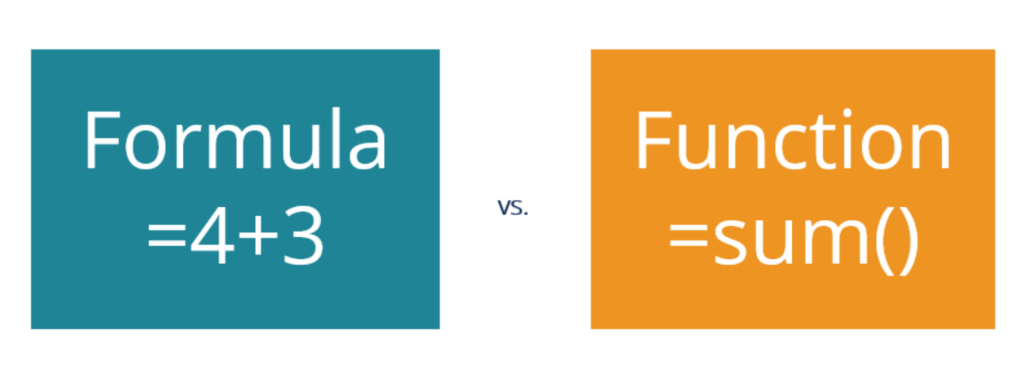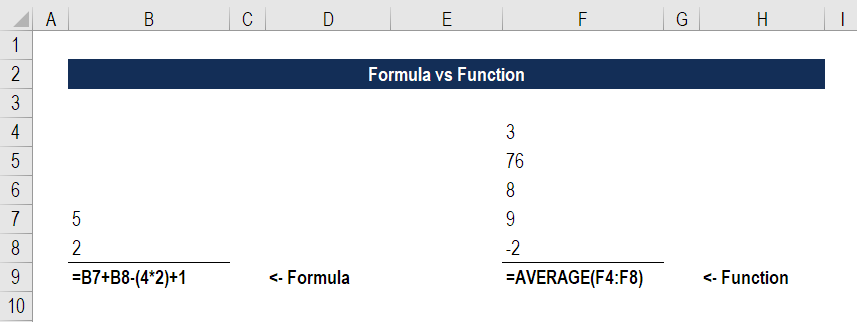Formula vs Function

Comparing Formula vs Function in Excel

What is a Formula vs Function in Excel?

A Formula is an equation designed by a user in Excel, while a Function is a predefined calculation in the spreadsheet application. This guide will walk you through a Formula vs Function in Excel so you know exactly what the similarities and differences are.

Excel allows users to perform simple calculations such as finding totals for a row or column of numbers. Formulas and functions can be useful in more complex situations, including calculating mortgage payments, solving engineering or math problems, and creating financial models.Examples of a Formula

When a user types an equals sign in a cell, they are starting to create a formula.

Examples of a formula include:

=4+3

=A3+C9

=B7+B8-(4*2)+1 [see screenshot below]

Examples of a Function

When a user types an equals sign followed by a predefined set of letters (or clicks on the Fx button in the formula bar), a function begins to be implemented.

=SUM(A3:A27)

=AVERAGE(F4:F8) [see screenshot below]

=NPV(0.12,A5:G5)Examples of a Formula and Function

It doesn’t just need to be a case of formula vs function. Users can combine the two to create even more powerful analysis.

Examples of the two combined are:

=SUM(A5:A8)/5

=AVERAGE(B3:B19)+25+SUM(1,2,3,5)

=NPV(0.12,A5:G5)*0.8

Formula vs Function in Practice

In common business practice, users use the terms formula and function almost interchangeably. From a communication and comprehension perspective, there’s not a big difference. Just know that technically, a function is a piece of code that executes a predefined calculation, and a formula is something you create yourself.

Thank you for reading this CFI guide to understanding Formula vs Function in Excel.

CFI is the official provider of the global Financial Modeling & Valuation Analyst (FMVA)™ certification program, designed to help anyone become a world-class financial analyst. To keep advancing your career, the additional resources below will be useful:

• List of Excel Shortcuts for PC & Mac
• List of Excel Functions